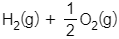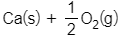# Problem: The enthalpy change, ΔH, for a few reactions are given. → H2O(l)                     ΔH = -286 kJ/molCa(s) + 2H+(aq) → Ca2+(aq) + H2(g)           ΔH = -544 kJ/molCaO(s) + 2H+(aq) → Ca2+(aq) + H2O(l)       ΔH = -193 kJ/molUsing Hess's law, calculate the heat of formation for CaO(s) using the following reaction. → CaO(s)              ΔH = ___ kJ/mol

###### FREE Expert Solution

Needed reaction: Ca(s) + 1/2 O2(g) → CaO(s)              ΔH = ___ kJ/mol

Reaction 1: H2(g) + 1/2 O2(g) → H2O(l)                         ΔH° = ‒286 kJ/mol

Reaction 2: Ca(s) + 2 H+(aq) → Ca2+(aq) + H2(g)           ΔH = ‒544 kJ/mol

Reaction 3: CaO(s) + 2 H+(aq) → Ca2+(aq) + H2O(l)       ΔH = ‒193 kJ/mol

For Reaction 1:

1/2 O2(g) → reactant side → no changes needed

Reaction 1: H2(g) + 1/2 O2(g) → H2O(l)                         ΔH° = ‒286 kJ/mol

For Reaction 2:

Ca(s)  → reactant side → no changes needed

87% (135 ratings)###### Problem Details

The enthalpy change, ΔH, for a few reactions are given.→ H2O(l)                     ΔH = -286 kJ/mol

Ca(s) + 2H+(aq) → Ca2+(aq) + H2(g)           ΔH = -544 kJ/mol

CaO(s) + 2H+(aq) → Ca2+(aq) + H2O(l)       ΔH = -193 kJ/mol

Using Hess's law, calculate the heat of formation for CaO(s) using the following reaction.→ CaO(s)              ΔH = ___ kJ/mol